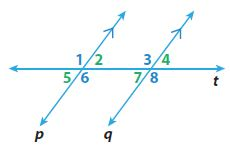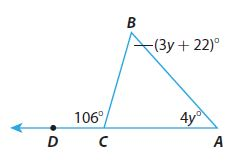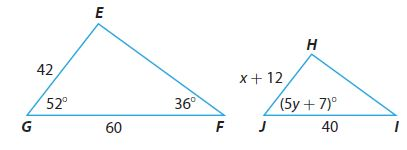Homework Explained - Math Practice 101Dear guest, you are not a registered member. As a guest, you only have read-only access to our books, tests and other practice materials.

As a registered member you can:

Registration is free and doesn't require any type of payment information. Click here to Register.
Go to page:
Chapter 11:Angle Relationships in Parallel Lines and Triangles; Model Quiz

11.1 Parallel Lines Cut by a Transversal

In the figure, line p$$\parallel$$line q. Find the measure of each angle if m$$\angle$$8 = 115°.•  m$$\angle7$$ = $$^°$$
•  m$$\angle 6$$ = $$^°$$
•  m$$\angle 1=$$ $$^°$$

11.2 Angle Theorems for Triangles

Find the measure of each angle.•  $$\text{m} \angle A =$$ $$^°$$
•  $$\text{m} \angle B=$$ $$^°$$
•  $$\text{m} \angle BCA=$$ $$^°$$

11.3 Angle-Angle Similarity

Triangle FEG is similar to triangle IHJ. Find the missing values.•  $$x=$$
•  y =
•  $$\text{m} \angle H =$$ $$^°$$

### ESSENTIAL QUESTION

• Question 10

How can you use similar triangles to solve real-world problems?

• Type below:

Yes, email page to my online tutor. (if you didn't add a tutor yet, you can add one here)